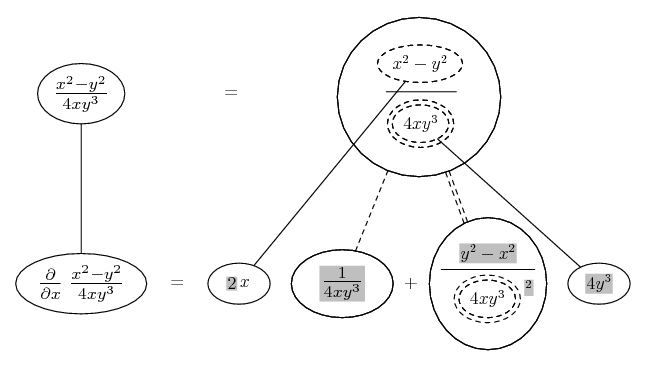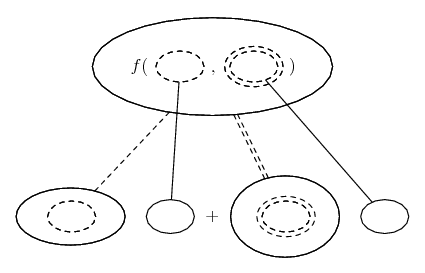# Thread: Help with Chain Rule problem (Multivariable Calc)

1. ## Help with Chain Rule problem (Multivariable Calc)

Hi there, I'm having a hard time solving this particular problem:

"Use appropriate forms of the chain rule to find the derivatives"
Let t = u/v; u = X^2 - y^2, v = 4xy^3

Find ∂t/∂x and ∂t/∂y

Now, the answer from the solutions manual is supposed to be
∂t/∂x = x^2 + y^2/4x^2y^3

∂t/∂y =y2 − 3x2/4xy4

Here's what I tried:
∂t/∂x = (2x - y^2)(4xy^3) - (x^2 - y^2)(4y^3)/ (4xy^3)^2

I couldn't come up with the right answer this way, I know I'm missing the chain rule here, according to the problem it has to be applied somewhere but I can't just figure out how. Can someone help me out with this?2.Originally Posted by TreizeHi there, I'm having a hard time solving this particular problem:

"Use appropriate forms of the chain rule to find the derivatives"
Let t = u/v; u = X^2 - y^2, v = 4xy^3

Find ∂t/∂x and ∂t/∂y

Now, the answer from the solutions manual is supposed to be
∂t/∂x = x^2 + y^2/4x^2y^3

∂t/∂y =y2 − 3x2/4xy4

Here's what I tried:
∂t/∂x = (2x - y^2)(4xy^3) - (x^2 - y^2)(4y^3)/ (4xy^3)^2

I couldn't come up with the right answer this way, I know I'm missing the chain rule here, according to the problem it has to be applied somewhere but I can't just figure out how. Can someone help me out with this?$\displaystyle \frac{\partial t}{\partial x}=\frac{\partial t}{\partial u}\frac{\partial u}{\partial x}+\frac{\partial t}{\partial v}\frac{\partial v}{\partial x}$.

Similarly,

$\displaystyle \frac{\partial t}{\partial y}=\frac{\partial t}{\partial u}\frac{\partial u}{\partial y}+\frac{\partial t}{\partial v}\frac{\partial v}{\partial y}$.

Can you take it from here?

3.Originally Posted by Chris L T521$\displaystyle \frac{\partial t}{\partial x}=\frac{\partial t}{\partial u}\frac{\partial u}{\partial x}+\frac{\partial t}{\partial v}\frac{\partial v}{\partial x}$.

Similarly,

$\displaystyle \frac{\partial t}{\partial y}=\frac{\partial t}{\partial u}\frac{\partial u}{\partial y}+\frac{\partial t}{\partial v}\frac{\partial v}{\partial y}$.

Can you take it from here?
Hehe, not really. Could you show how to obtain say $\displaystyle \frac{\partial t}{\partial u}\frac{\partial u}{\partial x}+$.? I think if I see how to partially solve it I can figure out the rest, it's just that by looking at the definitions I can't really solve the problem.

4. Hi - cherry-picking here and two days late but...

Just in case a picture helps...... If so, try the same for $\displaystyle \frac{\partial}{\partial y}\ \frac{x^2 - y^2}{4xy^3}$.... is the chain rule for two inner functions, i.e...

$\displaystyle \frac{d}{dx}\ f(u(x), v(x)) = \frac{\partial f}{\partial u} \frac{du}{dx} + \frac{\partial f}{\partial v} \frac{dv}{dx}$

As with...... the ordinary chain rule, straight continuous lines differentiate downwards (integrate up) with respect to x, and the straight dashed line similarly but with respect to the (corresponding) dashed balloon expression which is (one of) the inner function(s) of the composite expression.

Similar example at http://www.mathhelpforum.com/math-he...tml#post374503. Have shaded here though the expressions that were held constant on the way down (whilst differentiating with respect to some other expression).

_____________________________________

Don't integrate - balloontegrate!

Balloon Calculus Gallery

Balloon Calculus Drawing with LaTeX and Asymptote!

#### Search Tags

calc, chain, multivariable, problem, rule